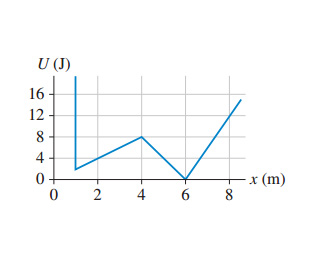# Problem: shows the potential energy of a 500 g particle as it moves along the x-axis. Suppose the particles mechanical energy is 12 J.Where are the particles turning points?What is the particles speed when it is at x = 4.0 m?What is the particles maximum speed?At what position or positions does this occur?Suppose the particle’s energy is lowered to 4.0 J. Can the particle ever be at x = 2.0 m? Suppose the particle’s energy is lowered to 4.0 J. Can the particle ever be at x = 4.0 m?

###### FREE Expert Solution
79% (320 ratings)
###### Problem Detailsshows the potential energy of a 500 g particle as it moves along the x-axis. Suppose the particles mechanical energy is 12 J.

Where are the particles turning points?

What is the particles speed when it is at x = 4.0 m?

What is the particles maximum speed?

At what position or positions does this occur?

Suppose the particle’s energy is lowered to 4.0 J. Can the particle ever be at x = 2.0 m?

Suppose the particle’s energy is lowered to 4.0 J. Can the particle ever be at x = 4.0 m?